Question

# In Millikan's experiment, an oil drop of radius 1.89 um and density 0.874 g/cm3 is suspended...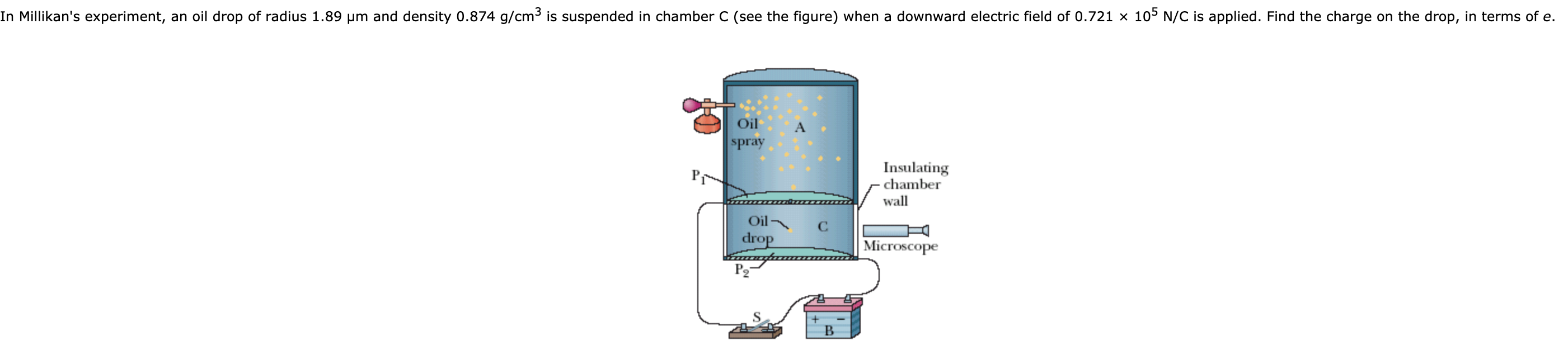In Millikan's experiment, an oil drop of radius 1.89 um and density 0.874 g/cm3 is suspended in chamber C (see the figure) when a downward electric field of 0.721 x 105 N/C is applied. Find the charge on the drop, in terms of e. Oil spray P1 Insulating chamber wall Oil drop С Microscope P2 B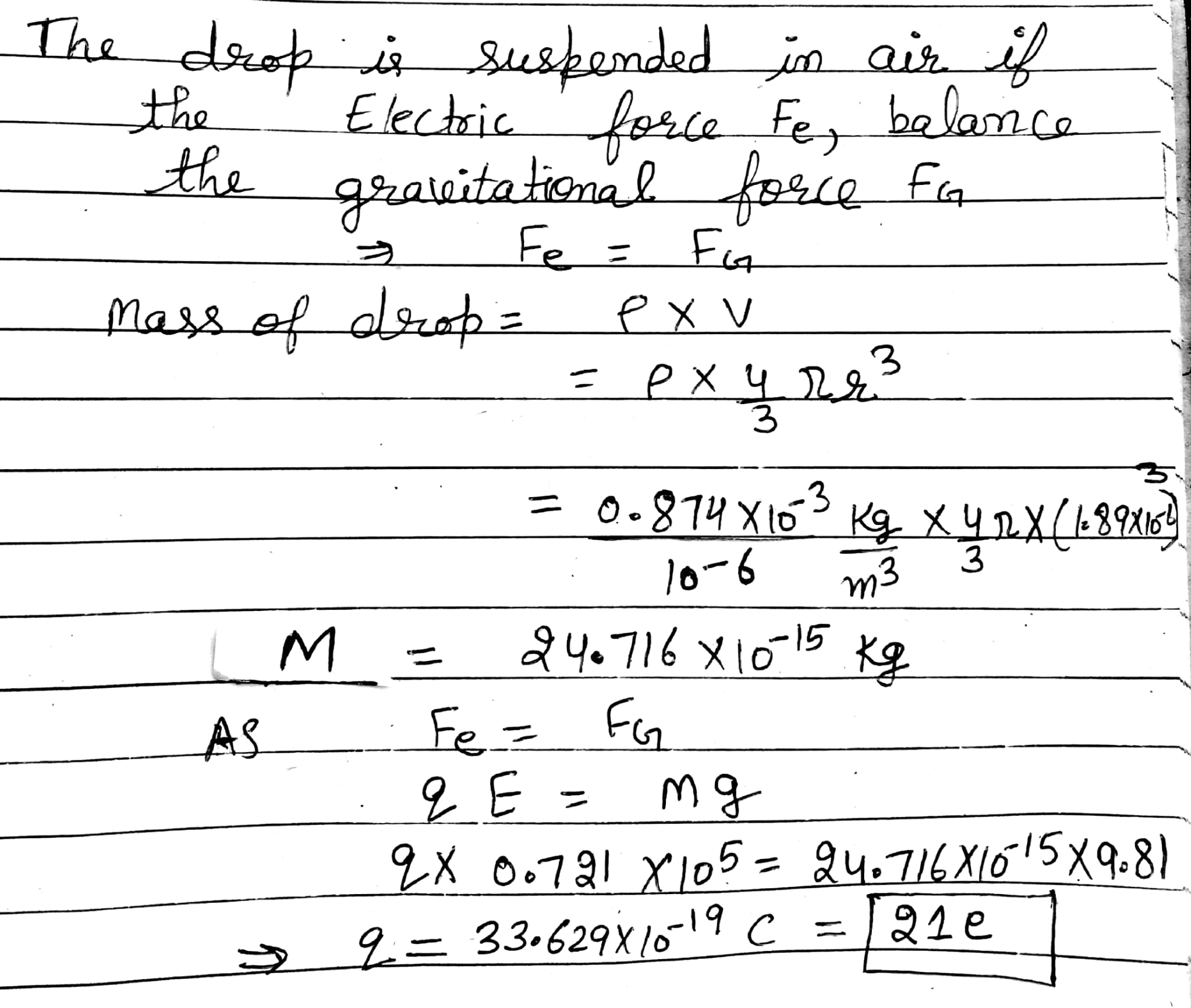#### Earn Coins

Coins can be redeemed for fabulous gifts.

Similar Homework Help Questions
• ### In Millikan's experiment, an oil drop of radius 2.012 µm and density 0.828 g/cm3 is suspended...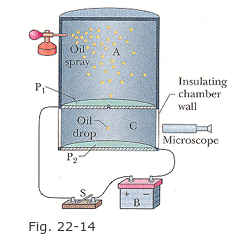In Millikan's experiment, an oil drop of radius 2.012 µm and density 0.828 g/cm3 is suspended in chamber C (Figure 22-14) when a downward-pointing electric field of 1.92* 10^5 N/C is applied. Find the charge on the drop, in terms of e. (Include the sign.) Thank youuuuu Oil spray Insulating chamber wall drop Microscope Fig. 22-14

• ### 1) In Millikan's experiment, an oil drop of radius 1.95 μm and density 0.857 g/cm3 is...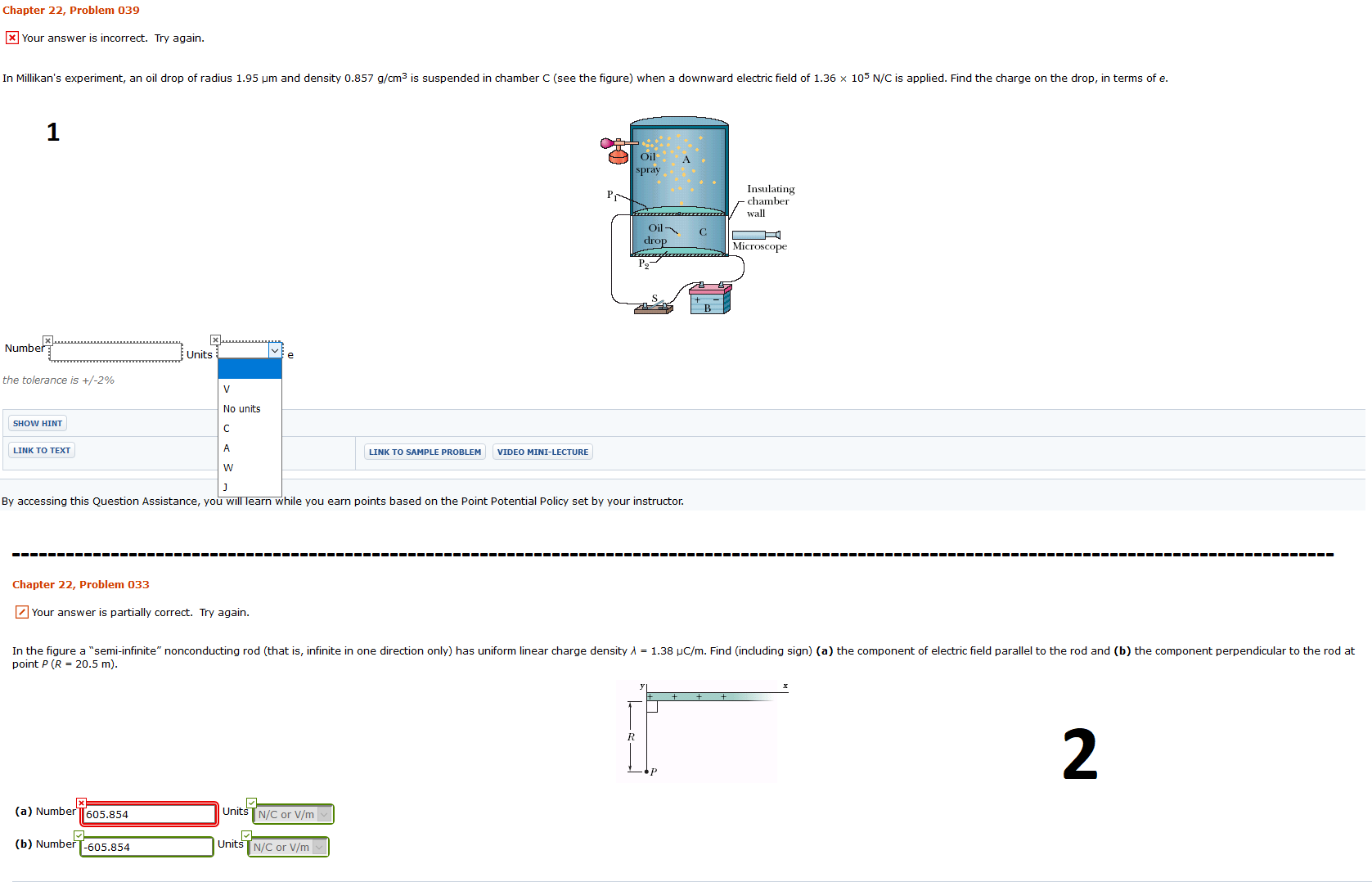1) In Millikan's experiment, an oil drop of radius 1.95 μm and density 0.857 g/cm3 is suspended in chamber C (see the figure) when a downward electric field of 1.36 × 105 N/C is applied. Find the charge on the drop, in terms of e. 2)In the figure a “semi-infinite” nonconducting rod (that is, infinite in one direction only) has uniform linear charge density λ = 1.38 μC/m. Find (including sign) (a) the component of electric field parallel to the...

• ### In Millikan's experiment, an oil drop of radius 1.70 mu m and density 0.859 g/cm^3 is...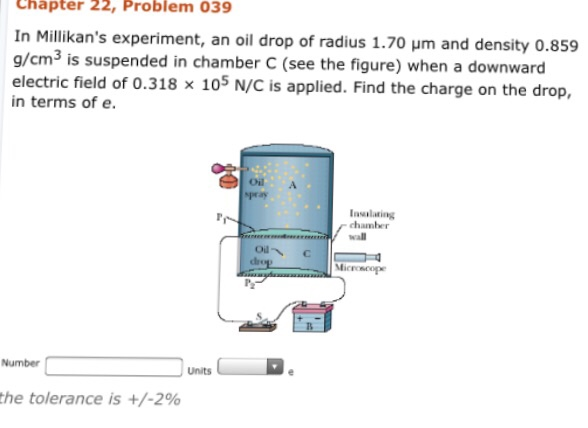In Millikan's experiment, an oil drop of radius 1.70 mu m and density 0.859 g/cm^3 is suspended in chamber C (see the figure) when a downward electric field of 0.318 times 10^5 N/C is applied. Find the charge on the drop, in terms of e. Number Units e

• ### In Millikan's oil drop experiment, a drop of radius r = 1.69 µm (1µm = 10-6m)...

In Millikan's oil drop experiment, a drop of radius r = 1.69 µm (1µm = 10-6m) and density 867 kg/m3 is suspended (against gravity) in a uniform electric field (Fe cancels Fg) when the drop has 4 excess electrons. What is the magnitude of the electric field (in N/C)? (Note that you can enter the full number or use the format 9.99E9 where "E9" represents 109). Enter only the numerical part of the answer (not the units).

• ### In Millikan's oil-drop experiment, one looks at a small oil drop held motionless between two plates....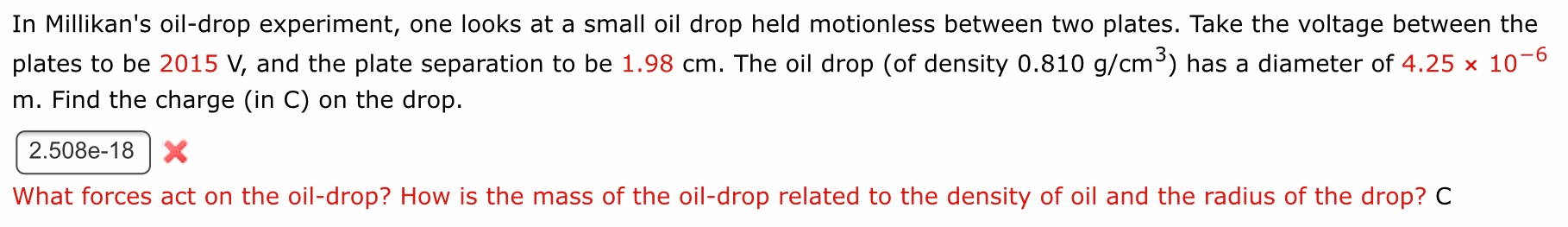In Millikan's oil-drop experiment, one looks at a small oil drop held motionless between two plates. Take the voltage between the plates to be 2015 V, and the plate separation to be 1.98 cm. The oil drop (of density 0.810 g/cm3) has a diameter of 4.25 x 10-6 m. Find the charge (in C) on the drop. 2.508e-18 X What forces act on the oil-drop? How is the mass of the oil-drop related to the density of oil and the...

• ### Millikan's oil drop experiment

In 1909 Robert Millikan was the first to find the charge of an electron in his now-famous oil drop experiment. In the experiment, tiny oil drops are sprayed into auniform electric field between a horizontal pair of oppositely charged plates. The drops are observed with a magnifying eyepiece, and the electric field is adjusted sothat the upward force on some negatively charged oil drops is just sufficient to balance the downward force of gravity. That is, when suspended, upward force...